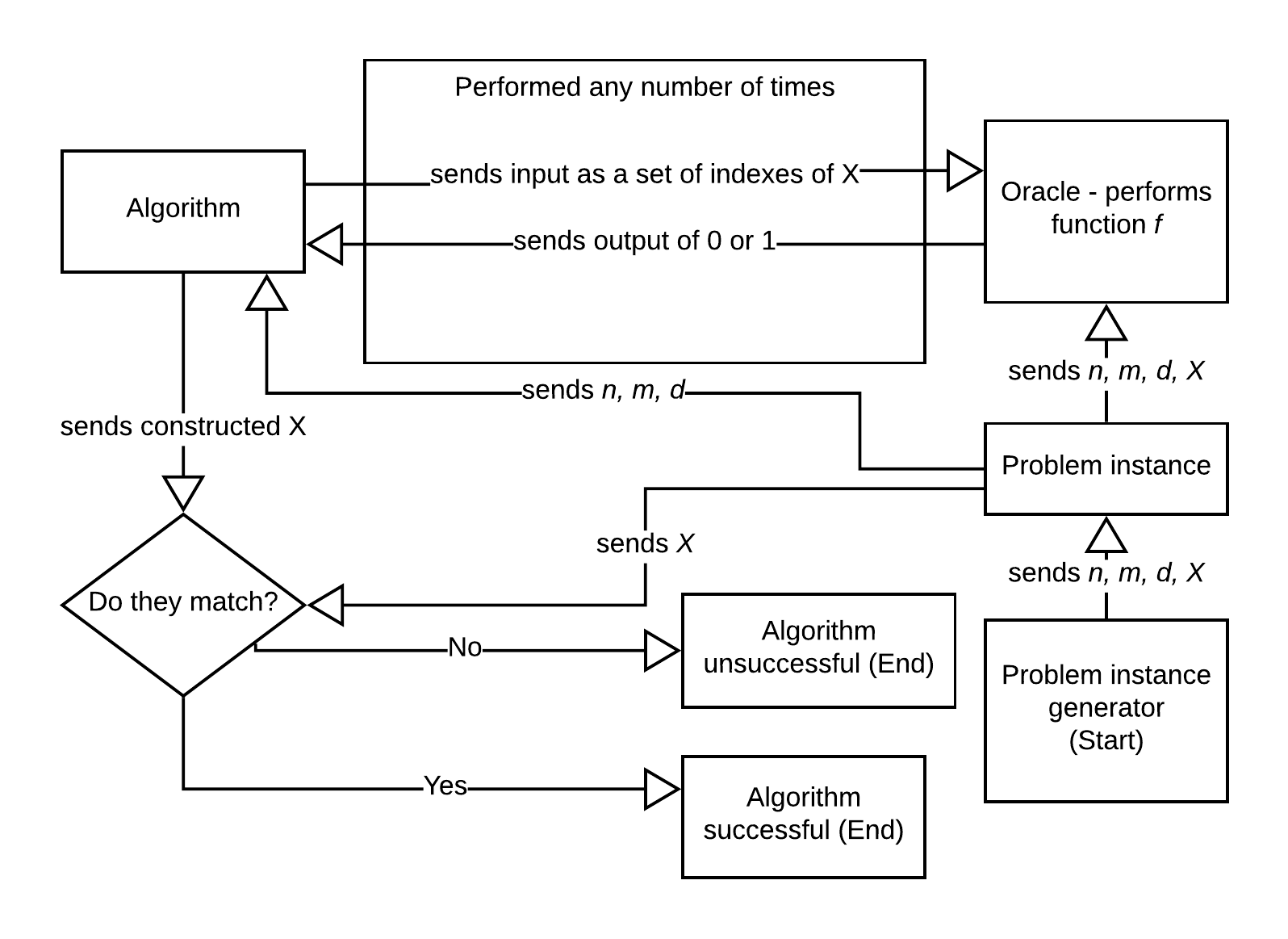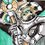# Infinite Xor Problem

Let $$m>n>d$$ be positive naturals and $$X=\langle x_{i}\mid i<m\rangle\in\{0,1\}^m$$. Define $$f_{X}:[m]^{n}\longrightarrow \{0,1\}$$ via $$f_{X}(i_{0},i_{1},\ldots,i_{n-1})=1$$ exactly in case $$|\{j<m\mid x_{i_{j}}=1\}|=d$$. Note, that the map $$X\in\{0,1\}^{m}\mapsto f_{X}$$ is not necessarily injective.

Now assume one has access to just $m,n,d,f$. How can one recover the list of all possible sequences $X$ via computational means, such that $f_{X}=f$? Theoretically one can go through (via lexical ordering) the list of all elements $Y\in\{0,1\}^{m}$ and compute each $f_{Y}$ and check if $f_{Y}=f$. But this will have a long average running time.

Construct an algorithm, $\mathcal{A}(\cdot,\cdot,\cdot,\cdot)$ which satisfies $\mathcal{A}(m,n,d,f)\subseteq\{0,1\}^{m}$ and $\forall{X\in\{0,1\}^{m}:~}X\in \mathcal{A}(m,n,d,f)\Leftrightarrow f_{X}=f$ for all $m>n>d$ and $f:[m]^{n}\longrightarrow\{0,1\}$. Further let $\mathcal{T}(m,n,d,f)$ be the total number of calls to the oracle $f$ during the execution of $\mathcal{A}(m,n,d,f)$ and $\overline{\mathcal{T}}(m,n,d):=\frac{1}{2^{|[m]^{n}|}}\sum_{f\in\{0,1\}^{[m]^{n}}}\mathcal{T}(m,n,d,f)$

which is effectively the average running time. Find an algorithm, $\mathcal{A}(\cdot,\cdot,\cdot,\cdot)$, such that $\overline{\mathcal{T}}(m,n,d)$ is minimised for all $m,n,d$, if this is at all possible.

There is a more relaxed version of this problem in which $d = 1$, and is a fixed value. This version may be more likely to have a polynomial time solution algorithm, or with an algorithm such that $\exists m\ \exists n\ \exists d \exists X: \mathcal{T}(m,n,d,f) < |X|$.

A basic flowchart of how the solution algorithm should function in practice:An example procedure:

The problem instance generator decides values $m = 3,\ n = 2, \ d = 1, \ X = \{0, 1, 1\}$. This information is sent to the oracle who can perform function $f$, and the values of $m$, $n$ and $d$ are sent to the algorithm.

The algorithm decides to call f(0, 1) to which the oracle returns 1 as it knows exactly one of $x_{0}$ and $x_{1}$ is 1 and the rest are 0. Then, the algorithm calls f(0, 2) which returns 1 and f(1, 2) which returns 0. Since $x_{1}$ and $x_{2}$ match and both are different to $x_{0}$, the algorithm can determine that $X = \{0, 1, 1\}$ or $X = \{1, 0, 0\}$. The algorithm sends both, and they compared with the $X$ sent by the problem instance generator. Since there is a match, the algorithm has done a successful run for one combination of $X$ for values $m = 3, n = 2, d = 1$.

Credit to R Mathe for helping rewrite and improve this.Note by Darcy Morgan
3 years, 2 months ago

This discussion board is a place to discuss our Daily Challenges and the math and science related to those challenges. Explanations are more than just a solution — they should explain the steps and thinking strategies that you used to obtain the solution. Comments should further the discussion of math and science.

When posting on Brilliant:

• Use the emojis to react to an explanation, whether you're congratulating a job well done , or just really confused .
• Ask specific questions about the challenge or the steps in somebody's explanation. Well-posed questions can add a lot to the discussion, but posting "I don't understand!" doesn't help anyone.
• Try to contribute something new to the discussion, whether it is an extension, generalization or other idea related to the challenge.

MarkdownAppears as
*italics* or _italics_ italics
**bold** or __bold__ bold
- bulleted- list
• bulleted
• list
1. numbered2. list
1. numbered
2. list
Note: you must add a full line of space before and after lists for them to show up correctly
paragraph 1paragraph 2

paragraph 1

paragraph 2

[example link](https://brilliant.org)example link
> This is a quote
This is a quote
    # I indented these lines
# 4 spaces, and now they show
# up as a code block.

print "hello world"
# I indented these lines
# 4 spaces, and now they show
# up as a code block.

print "hello world"
MathAppears as
Remember to wrap math in $$ ... $$ or $ ... $ to ensure proper formatting.
2 \times 3 $2 \times 3$
2^{34} $2^{34}$
a_{i-1} $a_{i-1}$
\frac{2}{3} $\frac{2}{3}$
\sqrt{2} $\sqrt{2}$
\sum_{i=1}^3 $\sum_{i=1}^3$
\sin \theta $\sin \theta$
\boxed{123} $\boxed{123}$

Sort by:

- 3 years, 2 months ago

That's okay! It's still reassuring to know that my idea is worth your time.

- 3 years, 2 months ago

Please clearly and formally redo the first set of bullet points and the paragraph before this. Your text is both mathematically and grammatically incomprehensible. For example

• What on Earth do you mean by pronumerals?
• You say determine all values of $X=\{x_{1},\ldots,x_{n}\}$ fine, I get it, that’s the representation form, such that $x$ maps to $\{0,1\}$. Sorry, what? Do you mean that each $x_{i}\in\{0,1\}$ or that each $x_{i}$ is a $\{0,1\}$-valued function, and if so with what domain?
• …for any $d$ in all $m$ This makes no sense in the context.

- 3 years, 2 months ago

Thanks for the feedback. While they are admittedly obvious mistakes now that I look back, I haven't had experience writing "mathematically" like this. I've rewritten it and taken away the example case to try and make it read smoother. Please let me know if it makes sense to you now or if I missed something else.

It seems important to keep both versions of the problem as the first version seems more likely to have a polynomial-time algorithm.

- 3 years, 1 month ago

Your current formulation is a lot clearer. Having both versions is absolutely fine. Remaining issues:

• I take it you really mean sequences $X=\langle x_{i}\mid i as opposed to sets, otherwise by the other condition there really is only one set, viz $X=\{0,1\}$.
• What exactly is supposed to be computed. Your problem is still frustratingly vague. Are we suppose to compute the set of all $n$-length $\{0,1\}$-sequences $X$ with certain properties? Or just one, or …?
• Let $\mathcal{A}$ be a given algorithm that returns upon valid input ($n>m>d$ in $\mathbb{N}^{+}$) an output $\mathcal{A}(n,m,d)\in\wp(\{0,1\}^{n})$. What exactly is supposed to go in the output? All $X$ such that […what…]? Right now, your definition just requires all $n$-length $\{0,1\}$-sequences $X$ such that $X$ contains all values and that’s it. There’s nothing to compute.

- 3 years, 1 month ago

Yes, I should be using sequences, I didn't realise there were angled brackets for that.

I've added a diagram and a more complete example to describe what I expect as a solution. What I am interested in is the lowest average case number of calls to f a successful algorithm needs and how it is affected by changing m, n and d.

- 3 years, 1 month ago

Okay, I think I understand. Your original description prove that it is possible to determine all values of $X$ is thus thoroughly misleading. The known inputs are $m$, $n$, $d$, the hidden inputs is the sequence $X$ itself. Moreover, the function is NOT to be executed as $f(x_{0},x_{1},\ldots,x_{m-1})$, as per the original description, but rather as $f(i_{0},i_{1},\ldots,i_{n-1})$ where $i_{0},i_{1},\ldots,i_{n-1}\in\{0,1,2,\ldots,m-1\}$ are distinct indices. The problem is thus:

Let $m>n>d$ be positive naturals and $X=\langle x_{i}\mid i. Define $f_{X}:[m]^{n}\longrightarrow \{0,1\}$ via $f_{X}(i_{0},i_{1},\ldots,i_{n-1})=1$ exactly in case $|\{j. Note, that the map $X\in\{0,1\}^{m}\mapsto f_{X}$ is not necessarily injective.

Now assume one has access to just $m,n,d,f$. How can one recover the list of all possible sequences $X$ via computational means, such that $f_{X}=f$? Theoretically one can go through (via lexical ordering) the list of all elements $Y\in\{0,1\}^{m}$ and compute each $f_{Y}$ and check if $f_{Y}=f$. But this will have a long average running time.

Construct an algorithm, $\mathcal{A}(\cdot,\cdot,\cdot,\cdot)$ which satisfies $\mathcal{A}(m,n,d,f)\subseteq\{0,1\}^{m}$ and $\forall{X\in\{0,1\}^{m}:~}X\in \mathcal{A}(m,n,d,f)\Leftrightarrow f_{X}=f$ for all $m>n>d$ and $f:[m]^{n}\longrightarrow\{0,1\}$. Further let $\mathcal{T}(m,n,d,f)$ be the total number of calls to the oracle $f$ during the execution of $\mathcal{A}(m,n,d,f)$ and

$\overline{\mathcal{T}}(m,n,d):=\frac{1}{2^{m}}\sum_{X\in\{0,1\}^{m}}\mathcal{T}(m,n,d,f_{X})$

which is effectively the average running time. Find an algorithm, $\mathcal{A}(\cdot,\cdot,\cdot,\cdot)$, such that $\overline{\mathcal{T}}(m,n,d)$ is minimised for all $m,n,d$, if this is at all possible.

Note: $[m]^{n}=[\{0,1,2,\ldots,m-1\}]^{n}=\{A\subseteq\{0,1,2,\ldots,m-1\}\mid |A|=n\}$, thus $|[m]^{n}|=\left(\begin{array}{c}[m]{l}m\\n\\\end{array}\right)$ elements.

- 3 years, 1 month ago

Very well written, and a tremendous thank you for rewriting it in an easier to understand format.

Now that I think about it, the algorithm might not need to compute all values of $X$ to find the average to a reasonable degree of accuracy, but yes that is why computing all of them seemed important at the time of writing.

A concrete purpose of this problem is investigating if it is possible that $\exists m\ \exists n\ \exists d\ \forall X: \overline{\mathcal{T}}(m,n,d) < |X|$ or the more loose condition $\exists m\ \exists n\ \exists d\ \exists X: \mathcal{T}(m,n,d,f) < |X|$ which could lead to a multiple compression algorithm where $n$, $m$ and $d$ are commonly known and the calls to $f_{X}$ as decided by the algorithm is stored inside a new $X$, which can be reduced using the algorithm again and so on.

Thanks again for all of your help.

- 3 years, 1 month ago

not a problem. Do you want to compute the average over all $f$ ie

$\overline{\mathcal{T}}(m,n,d):=\frac{1}{2^{|[m]^{n}|}}\sum_{f\in\{0,1\}^{[m]^{n}}}\mathcal{T}(m,n,d,f)$

or over all $X$ ie

$\overline{\mathcal{T}}(m,n,d):=\frac{1}{2^{m}}\sum_{X\in\{0,1\}^{m}}\mathcal{T}(m,n,d,f_{X})$

I came up with an algorithm. I’ll post it later. Not sure if it’s the most efficient, tho’.

- 3 years, 1 month ago

Over all $f$. The way I understand it currently, the average over all $X$ doesn't sound useful.

- 3 years, 1 month ago

There are two possibilities:

1. the problem is a kind of $d$-SAT problem: one is given (coded via $f$) knowledge of $m$ propositions and when for every $n$-Tuple exactly $d$ are true, and wishes to prove the existence of a model, satisfying these conditions. Then we need to compute the average over all $f$.

2. the problem is of the form: a hidden $X$ is given and we only see $f_{X}$. We are to compute a possible value of $X$. Then one needs to compute the average over all $X$.

My first algorithm so far is really inefficient: $\mathcal{T}(m,n,d,f)=m!/(m-n)!\sim m^{n}$. If $n,d$ are fixed then this is polynomial in $|X|$. If not, then this is exponential time (or worse!). The algorithm I employ is a simple recursion and doesn’t dynamically react to partial results, which is probably why it is not very fast. I need to think about this further.

Whilst your problem isn’t exactly $3$-SAT, there seem to be connections, and the latter is NP-hard, as far as I recall, so finding an efficient algorithm may be doomed. On the other hand, I’ve heard that replacing NP-problems by randomised counterparts may make more efficient algorithms possible.

- 3 years, 1 month ago

Do you really want the algorithm to compute all or to just find any one value of $X$?

- 3 years, 1 month ago